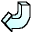Back# Flash Tools for Solving Equations

The following pages all require use of a plugin for Flash 4. If your browser needs one, you can download a copy from Macromedia's website.

### Linear Equations Practice

This Flash movie will help you solve three different types of equation.

Type 1: of the form x + 4 = 9

Type 2: of the form 5x - 1 = 14

Type 3: of the form 6x + 7 = 4x + 3

All you need to do is decide how much you need to add, subtract or divide each side of the equation. Click on the appropriate buttons, and the computer will perform the calculations for each step of your solution.

### Simultaneous Equation Solver

Type in the coefficients of x and y, along with the constants and this movie will solve any pair of simultaneous equations that can be solved.